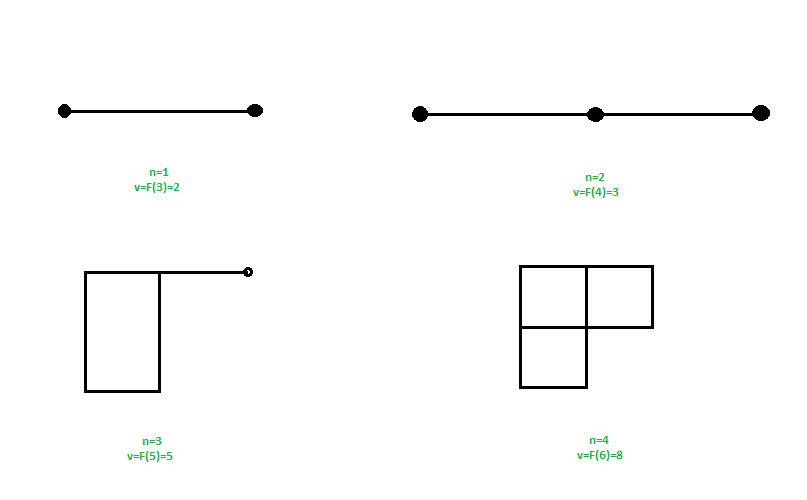Related Articles

# Fibonacci Cube Graph

• Difficulty Level : Easy
• Last Updated : 30 Apr, 2021

You are given input as order of graph n (highest number of edges connected to a node), you have to find the number of vertices in a Fibonacci cube graph of order n.
Examples :

```Input : n = 3
Output : 5
Explanation :
Fib(n + 2) = Fib(5) = 5

Input : n = 2
Output : 3```

A Fibonacci Cube Graph is similar to hypercube graph, but with a fibonacci number of vertices. In fibonacci cube graph only 1 vertex has degree n rest all has degree less than n.
Fibonacci cube graph of order n has F(n + 2) vertices, where F(n) is a n-th fibonacci number
Fibonacii series : 1, 1, 2, 3, 5, 8, 13, 21, 34……………….For input n as order of graph, find the corresponding fibonacci number at the position n + 2.
where F(n) = F(n – 1) + F(n – 2)
Approach : Find the (n + 2)-th fibonacci number.
Below is the implementation of above approach :

## C++

 `// CPP code to find vertices in a fibonacci``// cube graph of order n``#include``using` `namespace` `std;` `// function to find fibonacci number``int` `fib(``int` `n)``{``    ``if` `(n <= 1)``        ``return` `n;``    ``return` `fib(n - 1) + fib(n - 2);``}` `// function for finding number of vertices``// in fibonacci cube graph``int` `findVertices (``int` `n)``{``    ``// return fibonacci number for f(n + 2)``    ``return` `fib(n + 2);``}` `// driver program``int` `main()``{``    ``// n is the order of the graph``    ``int` `n = 3;``    ``cout << findVertices(n);``    ``return` `0;``}`

## Java

 `// java code to find vertices in a fibonacci``// cube graph of order n``public` `class` `GFG {``    ` `    ``// function to find fibonacci number``    ``static` `int` `fib(``int` `n)``    ``{``        ``if` `(n <= ``1``)``            ``return` `n;``        ``return` `fib(n - ``1``) + fib(n - ``2``);``    ``}``    ` `    ``// function for finding number of vertices``    ``// in fibonacci cube graph``    ``static` `int` `findVertices (``int` `n)``    ``{``        ``// return fibonacci number for f(n + 2)``        ``return` `fib(n + ``2``);``    ``}``        ` `    ``public` `static` `void` `main(String args[]) {``        ` `        ``// n is the order of the graph``        ``int` `n = ``3``;``        ``System.out.println(findVertices(n));``    ``}``}` `// This code is contributed by Sam007`

## Python3

 `# Python3 code to find vertices in``# a fibonacci cube graph of order n` `# Function to find fibonacci number``def` `fib(n):` `    ``if` `n <``=` `1``:``        ``return` `n``        ` `    ``return` `fib(n ``-` `1``) ``+` `fib(n ``-` `2``)` `# Function for finding number of``# vertices in fibonacci cube graph``def` `findVertices(n):` `    ``# return fibonacci number``    ``# for f(n + 2)``    ``return` `fib(n ``+` `2``)` `# Driver Code``if` `__name__ ``=``=` `"__main__"``:` `    ``# n is the order of the graph``    ``n ``=` `3``    ``print``(findVertices(n))` `# This code is contributed``# by Rituraj Jain`

## C#

 `// C# code to find vertices in a fibonacci``// cube graph of order n``using` `System;` `class` `GFG {``    ` `    ``// function to find fibonacci number``    ``static` `int` `fib(``int` `n)``    ``{``        ``if` `(n <= 1)``            ``return` `n;``        ``return` `fib(n - 1) + fib(n - 2);``    ``}``    ` `    ``// function for finding number of``    ``// vertices in fibonacci cube graph``    ``static` `int` `findVertices (``int` `n)``    ``{``        ` `        ``// return fibonacci number for``        ``// f(n + 2)``        ``return` `fib(n + 2);``    ``}``    ` `    ``// Driver code``    ``static` `void` `Main()``    ``{``        ` `        ``// n is the order of the graph``        ``int` `n = 3;``        ` `        ``Console.Write(findVertices(n));``    ``}``}` `// This code is contributed by Sam007`

## PHP

 ``

## Javascript

 ``
Output :
`5`

Note that the above code can be optimized to work in O(Log n) using efficient implementations discussed in Program for Fibonacci numbers

Attention reader! Don’t stop learning now. Get hold of all the important DSA concepts with the DSA Self Paced Course at a student-friendly price and become industry ready.  To complete your preparation from learning a language to DS Algo and many more,  please refer Complete Interview Preparation Course.

In case you wish to attend live classes with experts, please refer DSA Live Classes for Working Professionals and Competitive Programming Live for Students.

My Personal Notes arrow_drop_up